Courses

# RS Aggarwal Solutions: Whole Numbers Exercise - 3B Class 6 Notes | EduRev

## Class 6 : RS Aggarwal Solutions: Whole Numbers Exercise - 3B Class 6 Notes | EduRev

The document RS Aggarwal Solutions: Whole Numbers Exercise - 3B Class 6 Notes | EduRev is a part of the Class 6 Course Mathematics (Maths) Class 6.
All you need of Class 6 at this link: Class 6

Q.1. Fill in the blanks to make each of the following a true statements :
(i) 458 + 639 = 639 + ......
(ii) 864 + 2006 = 2006 + ......
(iii) 1946 + ...... = 984 + 1946
(iv) 8063 + 0 = ......

(v) 53501 + (574 + 799) = 574 + (53501 + ......)

Ans. (i) 458 + 639 = 639 + 458

(Commulative law)

(ii) 864 + 2006 = 2006 + 864

(Commulative law)

(iii) 1946 + 984 = 984 + 1946

(Commulative law)
(iv) 8063 + 0 = 8063

(Additive property of zero)
(v) 53501 + (574 + 799) = 574 + (53501 + 799)

(Associative law)

Q.2. Add the following numbers and check by reversing the order of the addents :
(i) 16509 + 114
(ii) 2359 + 548
(iii) 19753 + 2867
Ans.
(i) 16509 + 114 = 16623
Check : 16623 – 114 = 16509 which is given.
(ii) 2359 + 548 = 2907
Check : 2907 – 2359 = 548 which is given
(iii) 19753 + 2867 = 22620
Check : 22620 – 19753 = 2867 which is given

Q.3. Find the sum : (1546 + 498) + 3589. Also, find the sum : 1546 + (498 + 3589).
Are the two sums equal ? State the property satisfied.

Ans.
(1546 + 498) + 3589 = 2044 + 3589
= 5633
and 1546 + (498 + 3589) = 1546 + 4087
= 5633
Yes, the above two sum are equal.
The property used is associative law of addition.

Q.4. Determine each of the sums given below using suitable rearrangement.
(i) 953 + 707 + 647
(ii) 1983 + 647 + 217 + 353
(iii) 15409 + 278 + 691 + 422
(iv) 3259 + 10001 + 2641 + 9999
(v) 1 + 2 + 3 + 4 + 96 + 97 + 98 + 99
(vi) 2 + 3 + 4 + 5 + 45 + 46 + 47 + 48
Ans.
(i) 953 + 707 + 647
= (953 + 647) + 707
(by associative law)
= 1600 + 707 = 2307
(ii) 1983 + 647 + 217 + 353
= (1983 + 217) + (647 + 353)
= 2200 + 1000 = 3200
(iii) 15409 + 278 + 691 + 422

= (15409 + 691) + (278 + 422)
(by associative law)
= 16100 + 700 = 16800
(iv) 3259 + 10001 + 2641 + 9999
= (3259 + 2641) + (10001 + 9999)
(by associative law)
= 5900 + 20000 = 25900
(v) 1 + 2 + 3 + 4 + 96 + 97 + 98 + 99
= (1 + 99) + (2 + 98) + (3 + 97) + (4 + 96)
= (100 + 100) + (100 + 100)
= 200 + 200 = 400
(vi) 2 + 3 + 4 + 5 + 45 + 46 + 47 + 48
= (2 + 48) + (3 + 47) + (4 + 46) + (5 + 45)
= (50 + 50) + (50 + 50)
= 100 + 100 = 200

Q.5. Find the sum by short method :
(i) 6784 + 9999
(ii) 10578 + 99999
Ans. (i) 6784 + 9999 = (6784 – 1) + (9999 + 1)
(Adding and subtracting 1)
= 6783 + 10000 = 16783
(ii) 10578 + 99999
(Adding and subtracting 1)
= (10578 – 1) + (99999 + 1)
= 10577 + 100000 = 110577

Q.6. For any whole numbers a, b, c, is it true that (a + b) + c = a + (c + b) ? Give reasons.
Ans. Yes it is true, by the property of associative law of addition.

Q.7. Complete each one of the following magic squares by supplying the missing numbers :
(i)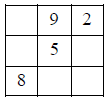(ii)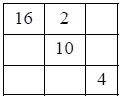(iii)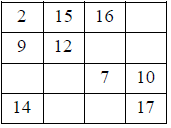(iv)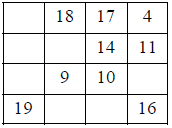Ans.
The magic squares given are completed as under :
(i)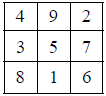In each row/column, the sum = 15
(ii)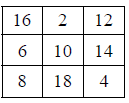In each row/column, the sum = 30
(iii)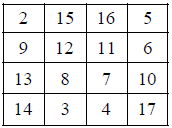In each rows/column, the sum = 38
(iv)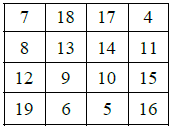In each row/column, the sum = 46

Q.8. Write (T) for true and (F) for false for each of the following statements :
(i) The sum of two odd numbers is an odd number.
(ii) The sum of two even numbers is an even number.
(iii) The sum of an even number and an odd number is an odd number.
Ans.
(i) The sum of two odd numbers is an odd number (F)
As sum of two odd numbers is alway an even number
(ii) The sum of two even number is an even number (T)
(iii) The sum of an even number and an odd number is an odd numebr (T)

Offer running on EduRev: Apply code STAYHOME200 to get INR 200 off on our premium plan EduRev Infinity!

## Mathematics (Maths) Class 6

191 videos|224 docs|43 tests

,

,

,

,

,

,

,

,

,

,

,

,

,

,

,

,

,

,

,

,

,

;